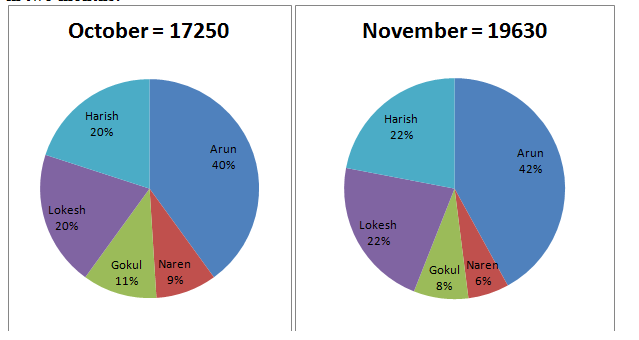# Quantitative Aptitude Questions (Data Interpretation) for SBI Clerk 2018 Day-3

## Quantitative Aptitude Questions (Data Interpretation) for SBI Clerk 2018 Day-3:

Dear Readers, SBI is conducting Online preliminary Examination for the recruitment of Clerical Cadre. preliminary Examination of SBI Clerk was scheduled from March 2018. To enrich your preparation here we have providing new series of Data Interpretation – Quantitative Aptitude Questions. Candidates those who are appearing in SBI Clerk Prelims Exam can practice these Quantitative Aptitude Average questions daily and make your preparation effective.

Click “Start Quiz” to attend these Questions and view Solutions

Directions(Q 1-5) The Table below shows percentage of marks obtained by 5 Students in different subjects:-

 Students Mathematics ( 200) Science (150) English (120) Social Science ( 160) Sumit 67 60 70 50 Rohit 84 50 75 60 Amit 74 64 60 80 Arnab 75 76 80 45 Kishore 72 54 55 80
1. Find the average marks obtained by Rohit in all the subjects.
1. 107.25
2. 120
3. 139
4. 132
5. None of these
1. The marks obtained by Arnab in English and Social Science is how much percent more/ less than the Marks obtained by Sumit in Mathematics and English?(Approx.)
1. 24
2. 25
3. 20
4. 23
5. None of these
1. If the total marks obtained by Amit in Mathematics, Science,English, Social Science and E.V.S is 560 then find by how much the marks obtained in E.V.S is less than the marks obtained in Mathematics?
1. 25
2. 30
3. 23
4. 21.62
5. None of these
1. Find the percentage of total marks obtained by Kishore in all the subjects.
1. 45
2. 78
3. 66.5
4. 56.25
5. None of these
1. Find the average marks obtained by all the students in Science.(Approx.)
1. 85
2. 89
3. 95
4. 91
5. None of these

Directions (Q. 6-10): The given pie-charts show the savings of different friends in two months:1. The savings of Harish in November is what per cent more than the savings of Gokul in October?
1. 130.25%
2. 156.91%
3. 127.59%
4. 125.74%
5. 120.56%
1. The savings of Lokesh in November is what per cent more than the savings of Harish in October? (Rounded off the nearest percentage)
1. 15%
2. 45%
3. 35%
4. 25%
5. 30%

1. What is the average savings of Naren and Lokesh in November and Arun and Harish in October? (Rounded off the nearest digit)
1. 3680
2. 3740
3. 4080
4. 3810
5. 3960
1. What is the percentage increase in savings of Arun from October to November?
1. 19.49%
2. 17.56%
3. 20.85%
4. 18.21%
5. 15.58%
1. The total amount of savings of Naren and Harish together in both the months is?
1. 11500
2. 10500
3. 10650
4. 11250
5. 10900

Directions(Q 1-5)

Total Marks obtained by Rohit in all the subjects = 168+75+ 90+ 96= 429

Average marks obtained by Rohit = 429/4= 107.25

Total Marks obtained by Arnab in English and Social Science= 96+ 72= 168

Total Marks obtained by Sumit in Mathematics and English= 134+ 84= 218

Arnab’s marks to be less than Arnab = 50

Required percentage= 50/218*100= 23

Total marks obtained by Amit in 5 subjects= 560

Marks obtained by Amit in E.V.S= 560 – (148+96+72+128) = 116

Marks obtained in E.V.S is less than mathematics= 148-116= 32

Required percentage= 32/148*100= 21.62

Total marks obtained by Kishore= 144+ 81+66+128= 419

Maximum Marks= 630

Required Percentage= 419/630*100= 66.50%

Total marks obtained by all the students in Science= 90+75+96+114+81= 456

Average marks obtained by them= 456/5= 91

Directions (Q. 6-10):

Savings of Harish in November = 19630 × 22 / 100 = 4318.6

Savings of Gokul in October = 17250 × 11 / 100 = 1897.5

Required %

= ((4318.6 – 1897.5) / 1897.5) × 100

= (2421.1/1897.5) × 100

= 127.59%

Savings of Lokesh in November

= 19630 × 22 / 100 = 4319

Savings of Harish in October

= 17250 × 20 / 100 = 3450

Required % = (4319 – 3450) / 3450 × 100

= 25%

Average = {(19630 × 6 / 100 + 19630 × 22 / 100 + 17250 × 40 / 100 + 17250 × 20 / 100) / 4}

= 1178 + 4319 + 6900 + 3450 / 4

= 15487 / 4

= 3961.75

= 3960

Savings of Arun in October = 17250 × 40 / 100 = 6900

Savings of Arun in November = 19630 × 42 / 100 = 8245

Required % = (8245 – 6900) / 6900 × 100

= 19.49%

Total savings of Naren and Harish together in both the months

In October = 17250 × 9/100 + 17250 × 20/100

= 1553 + 3450

= 5003

In November = 19630 × 6 / 100 + 19630 × 22/100

= 1178 + 4319

= 5497

Total = 5003 + 5497 = 10500